## LCM and HCF

#### LCM and HCF

1. If the product of three consecutive numbers is 210 then sum of the smaller number is :

1.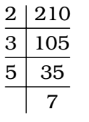∴ 210 = 2 × 3 × 5 × 7 = 5 × 6 × 7
Here , 5 and 6 are smaller numbers .

##### Correct Option: D∴ 210 = 2 × 3 × 5 × 7 = 5 × 6 × 7
Here , 5 and 6 are smaller numbers .
∴ Sum of smaller numbers = 5 + 6 = 11

1. The number between 4000 and 5000 that is divisible by each of 12, 18, 21 and 32 is

1. We find LCM of 12, 18 , 21 and 32.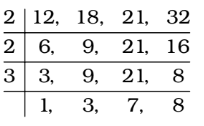∴ LCM of 12, 18 , 21 and 32 = 2 × 2 × 3 × 3 × 7 × 8 = 2016

##### Correct Option: B

We find LCM of 12, 18 , 21 and 32.∴ LCM of 12, 18 , 21 and 32 = 2 × 2 × 3 × 3 × 7 × 8 = 2016
∴ Required number = 2016 × 2 = 4032

1. A number between 1000 and 2000 which when divided by 30, 36 and 80 gives a remainder 11 in each case is

1. We find LCM of 30, 36 and 80.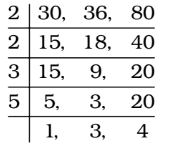∴ LCM = 2 × 2 × 3 × 3 × 4 × 5 = 720

##### Correct Option: A

We find LCM of 30, 36 and 80.∴ LCM = 2 × 2 × 3 × 3 × 4 × 5 = 720
∴ Required number = 2 × 720 + 11 = 1440 + 11 = 1451

1. The smallest five digit number which is divisible by 12,18 and 21 is :

1. Firstly , we find the LCM of 12, 18 and 21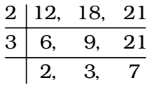∴ LCM of 12, 18 and 21 = 2 × 3 × 2 × 3 × 7 = 252

##### Correct Option: C

Firstly , we find the LCM of 12, 18 and 21∴ LCM of 12, 18 and 21 = 2 × 3 × 2 × 3 × 7 = 252
Of the options, 10080 ÷ 252 = 40
Hence , required answer is 10080 .

1. Let p be the smallest number, which when added to 2000 makes the resulting number divisible by 12, 16, 18 and 21. The sum of the digits of p is

1. We find the LCM of 12 , 16 , 18 and 21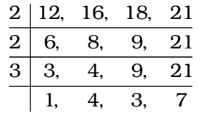∴ LCM = 2 × 2 × 3 × 4 × 3 × 7 = 1008
2 Multiple of 1008 = 1008 × 2 = 2016
∴ Required number = 2016 – 2000 = 16 = p

##### Correct Option: A

We find the LCM of 12 , 16 , 18 and 21∴ LCM = 2 × 2 × 3 × 4 × 3 × 7 = 1008
2 Multiple of 1008 = 1008 × 2 = 2016
∴ Required number = 2016 – 2000 = 16 = p
∴ Sum of digits of p = 1 + 6 = 7